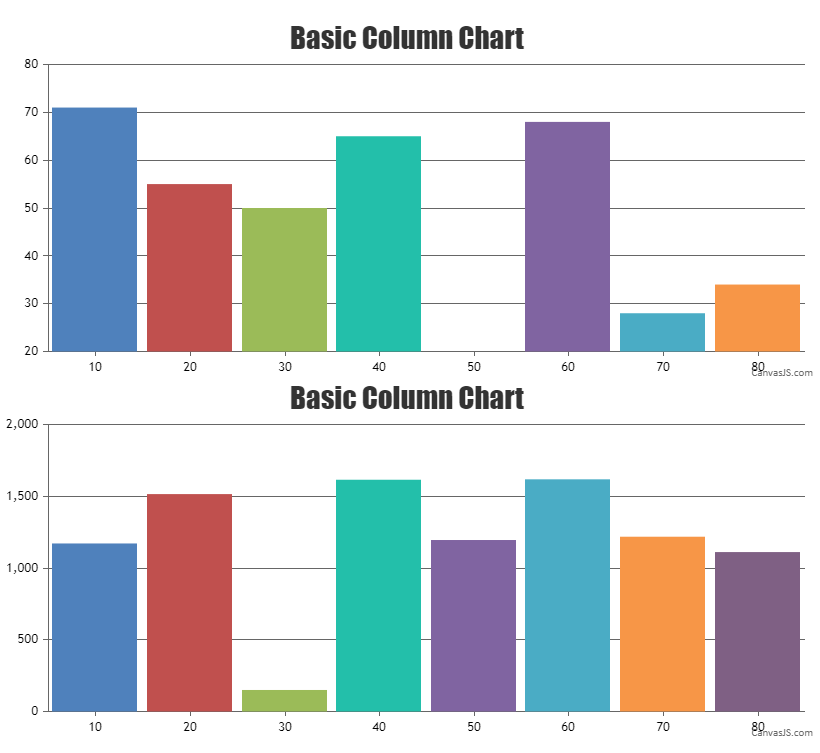Home Forums Chart Support Synchronized Charts Reply To: Synchronized Charts

#34448

You can align y-axis across multiple charts by setting the margin property. The margin value has to be calculated based on the bounds of y-axis as shown in the code below –

``````var axisYBoundMax = 0;
for (var i=0; i<charts.length; i++) {
axisYBoundMax = Math.max(axisYBoundMax, charts[i].axisY.bounds.x2);
}

for(var i = 0; i < charts.length; i++) {
charts[i].axisY.set("margin", axisYBoundMax - (charts[i].axisY.bounds.x2 - charts[i].axisY.bounds.x1));
} ``````

Please take a look at this JSFiddle for complete working code.—-
Manoj Mohan
Team CanvasJS